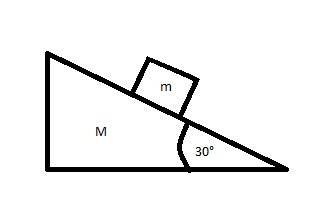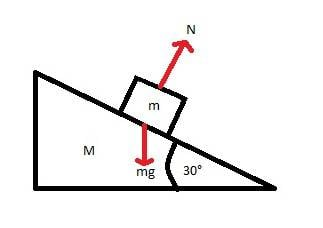# Block on accelerating wedge

Rococo

## Homework Statement

A wedge of mass M rests on a horizontal table. A block of mass m rests on the wedge.

http://imgur.com/cS02MvM

(a) What horizontal acceleration, a, must M have relative to the table to keep the block stationary relative to the wedge, assuming no friction?

(b) What horizontal force F must be applied to the system to achieve this result, assuming a frictionless table top?

F=(m+M)a

## The Attempt at a Solution

http://imgur.com/etif1qn

All I have so far is this diagram as I don't understand the concept involved. Its not clear to me why the horizontal acceleration of the wedge could keep the block stationary. Also, I'm not sure whether this acceleration would be to the left or to the right.

Also I have read through other similar threads but still can not understand the problem.

voko

## Homework Statement

A wedge of mass M rests on a horizontal table. A block of mass m rests on the wedge.(a) What horizontal acceleration, a, must M have relative to the table to keep the block stationary relative to the wedge, assuming no friction?

(b) What horizontal force F must be applied to the system to achieve this result, assuming a frictionless table top?

F=(m+M)a

## The Attempt at a SolutionAll I have so far is this diagram as I don't understand the concept involved. Its not clear to me why the horizontal acceleration of the wedge could keep the block stationary. Also, I'm not sure whether this acceleration would be to the left or to the right.

Also I have read through other similar threads but still can not understand the problem.

Start by writing the FBD equations. Note that for mass m to be stationary with respect to the wedge, its vertical acceleration must be zero, and it horizontal acceleration must be equal to that of M.

Homework Helper
Gold Member
Its not clear to me why the horizontal acceleration of the wedge could keep the block stationary.
It does not keep the block stationary. It keeps it stationary relative to the wedge.
To get the concept, imagine you have a block of ice on a flat tray. If you pull the tray swiftly to one side the ice block will be left behind. Now make the tray slightly wedge shaped so that the ice has to rise slightly in order to slide off. It will still slide off if you jerk the tray fast enough. So increase the slope a bit more....

nil1996
Start by writing Free body diagrams.Create equations applying
ƩFx=max &
ƩFy=may

This will give all the needed equations.

Rococo
Start by writing the FBD equations. Note that for mass m to be stationary with respect to the wedge, its vertical acceleration must be zero, and it horizontal acceleration must be equal to that of M.

Here's what I got:

Ncos30° = mg

Nsin30° = F = (m+M)a

Is this right?

Rococo
It does not keep the block stationary. It keeps it stationary relative to the wedge.
To get the concept, imagine you have a block of ice on a flat tray. If you pull the tray swiftly to one side the ice block will be left behind. Now make the tray slightly wedge shaped so that the ice has to rise slightly in order to slide off. It will still slide off if you jerk the tray fast enough. So increase the slope a bit more....

What's the reason this happens? Is it just as a result of the inertia of the block?

Homework Helper
Gold Member
Here's what I got:

Ncos30° = mg

Nsin30° = F = (m+M)a

Is this right?
The first equation, yes. But is F above the force applied to the wedge, as in the question? Why would that be equal to N sin 30? What body did you study an FBD of to obtain it?

Homework Helper
Gold Member
What's the reason this happens? Is it just as a result of the inertia of the block?
Yes. For the block to accelerate as fast as the wedge it needs a sufficient horizontal force. If the slope is too low or the friction inadequate then it won't keep up.

Rococo
The first equation, yes. But is F above the force applied to the wedge, as in the question? Why would that be equal to N sin 30? What body did you study an FBD of to obtain it?

Yes, F is the force applied to the wedge.

I realise F is not equal to Nsin30° now. The horizontal force acting on the block is Nsin30°. The horiztonal force acting on the wedge is F.

As voko said, the horizontal acceleration of the block must be equal to the horizontal acceleration of the wedge. So, since acceleration is force divided by mass I get this:

(Nsin30°)/m = F/(m+M)

Homework Helper
Gold Member
Yes, F is the force applied to the wedge.

I realise F is not equal to Nsin30° now. The horizontal force acting on the block is Nsin30°. The horiztonal force acting on the wedge is F.

As voko said, the horizontal acceleration of the block must be equal to the horizontal acceleration of the wedge. So, since acceleration is force divided by mass I get this:

(Nsin30°)/m = F/(m+M)
Yes.

Rococo
Yes.

Using the two equations:

(Nsin30°)/m = F/(m+M)
Ncos30° = mg

I was able to rearrange to get the following:

F/(m+M) = gtan30°
a = gtan30°
F = (m+M)gtan30°

Homework Helper
Gold Member
Using the two equations:

(Nsin30°)/m = F/(m+M)
Ncos30° = mg

I was able to rearrange to get the following:

F/(m+M) = gtan30°
a = gtan30°
F = (m+M)gtan30°
Looks good.